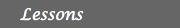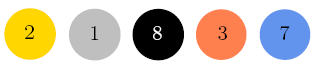MATH EXPLORERS' CLUB Cornell Department of Mathematics

# Billiards and Puzzles

 Introduction1. Rectangular Billiards 2. Circular/Elliptical Tables 3. Triangular Billiards 4. Puzzles 5. Two-Hole Billiards References## Billiards and PuzzlesIn this module we describe some of the mathematics behind billiards. We will first look at the shape of the table: rectangle, square, circle, ellipse, etc. In general the table can have any form, from very simple to very complicated. We will also explain some puzzles related to billiards, some of them having unusual solutions. Even if the rules of billiards are simple, the solution of these puzzles can become complex, depending on the situation.

This work was made possible due to a grant from the National Science Foundation.

 Top Next lesson